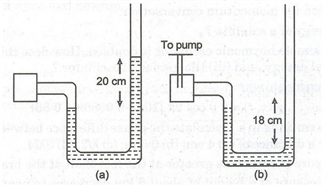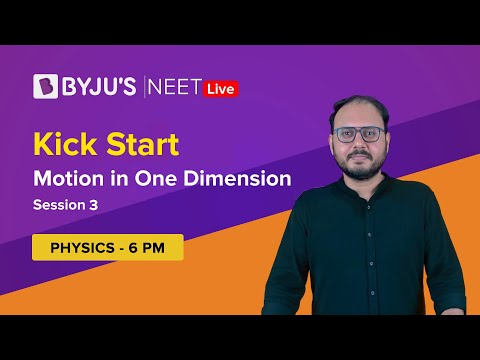# Important 5 marks Question For Physics Class 11

The Central Board of Secondary Education known as CBSE is one of the popular educational boards in the country. The board is known for its well-structured question paper and comprehensive syllabus. CBSE conducts the 10th and 12th board exams every year, mostly in the month of march. Students who are going to appear in the class 11 exams must prepare well, as the concepts in the 11th standard are very important for the class 12 board exams.

Physics is a very important subject for 11th standard science stream students. To get into a good college, students must score well in physics. Many students fear the subject and are unable to score well in their board exam. But if a student understands the concepts properly the subject can be very easy. One of the best ways to prepare for this subject is by practising physics questions. The important questions for physics class 11th are given here to help students prepare for their exams more effectively.

## Important 5 marks Question For Physics Class 11

The 5 marks questions in the physics paper are one of the most scoring section in the exam. The important 5 marks question for physics class 11th are given here. The questions are prepared by subject experts according to the latest CBSE syllabus of class 11 physics.

The Important 5 marks Question for Physics Class 11th is given below.

Question 1- Define electric flux. Write it’s S.I unit. State and explain Gauss’s law. Find out the outward flux to a point charge +q placed at the center of a cube of side ‘a’. Why is it found to be independent of the size and shape of the surface enclosing it? Explain

Question 2- (a) In Young’s double-slit experiment, deduce the conditions for obtaining constructive and destructive interference fringes. Hence, deduce the expression for the fringe width.

(b) Show that the fringe pattern on the screen is actually a superposition of slit diffraction from each slit.

(c)What should be the width of each slit to obtain 10 maxima of the double-slit pattern within the central maximum of the single slit pattern, for the green light of wavelength 500 nm, if the separation between two slits is 1 mm?

Question 3-(a) Draw the magnetic field lines due to a circular loop area

$$\begin{array}{l}\vec{A}\end{array}$$
carrying current I. Show that it acts as a bar magnet of the magnetic moment
$$\begin{array}{l}\vec{m}= I \vec{A}\end{array}$$

(b)Derive the expression for the magnetic field due to a solenoid of length ‘2l’, radius ‘A’ having ’n’ number of turns per unit length and carrying a steady current ‘I’ at a point on the axial line, distance ‘r’ from the center of the solenoid. How does this expression compare with the axial magnetic field due to a bar magnet of magnetic moment ‘m’?

Question 4-

(a) Deduce the expression for the potential energy of a system of two charges

$$\begin{array}{l}q_{1}\end{array}$$
and
$$\begin{array}{l}q_{2}\end{array}$$
located
$$\begin{array}{l}r_{1}\end{array}$$
and
$$\begin{array}{l}r_{1}\end{array}$$
respectively, in an external electric field.

(b) Three-point charges, +Q, + 2Q and –  3Q are placed at the vertices of an equilateral triangle ABC of side l. If these charges are displaced to the mid-point

$$\begin{array}{l}A_{1}\end{array}$$
,
$$\begin{array}{l}B_{1}\end{array}$$
and
$$\begin{array}{l}C_{1}\end{array}$$
respectively, find the amount of the work done in shifting the charges to the new locations.Question 5-(a) Two thin convex lenses

$$\begin{array}{l}L_{1}\end{array}$$
and
$$\begin{array}{l}L_{2}\end{array}$$
of focal lengths
$$\begin{array}{l}f_{1}\end{array}$$
and
$$\begin{array}{l}f_{2}\end{array}$$
respectively, are placed coaxially in contact. An object is placed at a point beyond the focus of lens
$$\begin{array}{l}L_{1}\end{array}$$
. Draw a ray diagram to show the image formation by the combination and hence derive the expression for the focal length of the combined system.

(b) A ray PQ incident on the face AB of a prism ABC, as shown in the figure below, emerges from the face AC such that AQ=AR.Draw the ray diagram showing the passage of the ray through the prism. If the angle of the prism is

$$\begin{array}{l}60^{\circ}\end{array}$$
and the refractive index of the material of prism is
$$\begin{array}{l}\sqrt{3}\end{array}$$
, determine the angle of incidence and angle of deviation.

Question 6-(a) Find relation for temperature gradient.

(b) Show the expression for heat flow through the compound wall.

Question 7-Prove the theorem of perpendicular axis.

Question 8-Derive the relations-

(i)

$$\begin{array}{l}v^{2} = u^{2} + 2as\end{array}$$

(ii)

$$\begin{array}{l}v = u + at\end{array}$$

(iii)

$$\begin{array}{l}S = ut + \frac{1}{2}at^{2}\end{array}$$

Question 9-Prove the theorem of parallel axis.

Question 10-(a) Derive an expression to find the specific heat with the help of calorimeter.

(b) Find the coefficient of thermal conductivity.

Question 11-(a) In a car race, car A takes a time t less than car B and finishes the finishing point with a velocity v more than that of the car B. Assuming that the cars start from rest and travel with, show constant accelerations

$$\begin{array}{l}a_{1}\end{array}$$
and
$$\begin{array}{l}a_{2}\end{array}$$
respectively, show that
$$\begin{array}{l}v = \sqrt{a_{1}.a_{2}}\end{array}$$
.

(b) The displacement of a particle moving in one dimension under the action of a constant force is related to the time t by the equation

$$\begin{array}{l}t = \sqrt{x + 3}\end{array}$$
, where x is in meter and t is in second. Find the displacement of the particle when its velocity is zero.

Question 12-(a) Determine the numerical value of R and KB.

(b) Obtain the relation between degrees of freedom of gas and ratio of two principal specific heat of the gas.

Question 13– A nanometer reads the pressure of the gas in an enclosure as shown in figure (a). When a pump removes some gas, the manometer reads as in figure (b). The liquid used in the manometers is mercury and the atmospheric pressure is 76 cm of mercury.(i) Give the absolute and gauge pressure of the gas in the enclosure for cases (a) and (b) in units of cm of mercury.

(ii) How would the levels change in case (b) if 13.6 cm of water (immiscible with mercury) is poured into the right limb of the manometer? (Ignore the small change in volume of the gas).

Question 14-(a) State and explain Charles’  law.

(b) State and derive the ideal gas equation.

Question 15-Explain why:

(a) To keep a piece of paper horizontal, you should blow over, not under it.

(b) When we try to close a water tap with our fingers, fast jets of water gush through the openings between our fingers.

(c) The size of the needle of a syringe controls the flow rate better than the thumb pressure exerted by a doctor while administering an injection.

(d) A fluid flowing out of a small hole in a vessel results in a backward thrust on the vessel.

(e) A spinning cricket ball in air does not follow a parabolic trajectory.

Question 16-a) Prove work-energy theorem for a variable force.

(b) Two protons are brought towards each other. Will the potential energy of the system decrease or increase? If a proton and an electron be brought nearer, then?

Question 17-A block initially at rest breaks into two parts of masses in the ratio 2 : 3. The velocity of smaller part is (81 + 6:1) m/s. Find the velocity of the bigger part.

In a nerve impulse, about 10 neutrons are fired. If energy associated with d is a charge of a single neutron is

$$\begin{array}{l}10^{-10}\end{array}$$
J, estimate the energy used.

Question 18-State and prove Bernoulli’s principle for the flow of non-viscous fluids.

Question 19-Prove that the coefficient of restitution of a perfectly elastic collision in one dimension is unity.

Question 20-(a) State and prove impulse-momentum theorem.

(b) Define coefficient of friction and angle of friction and hence derive a relation between them.

## This episode of the Kick Start series deep dives into the topic of Motion in One Dimension.Stay tuned with BYJU’S to learn various concepts of Physics with the help of attractive video lessons.